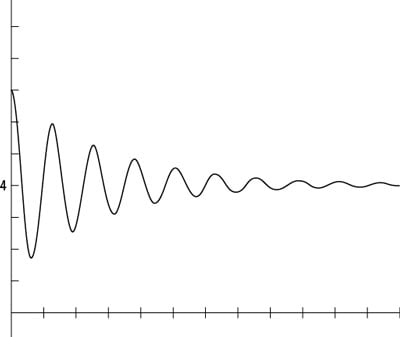##### Trigonometry For DummiesThe graph of a trigonometry function can be useful for showing a progression over time, such as in a model for the height of an object attached to a spring. The same pattern doesn’t occur over and over as in the sine function, but this is a great example of a trig function at work.The height of a bouncing object attached to a spring.

The equation H (t) = 3(0.7)t cos 5t + 4 represents the height of an object attached to a spring, where t is the amount of time that has passed — usually in seconds. The equation has a trigonometry function multiplied by an exponential function. When you first release the spring, the object hits a height of about 7 feet. It jumps up and down, finally settling in at about 4 feet high, as shown in the figure.

You can probably come up with a similar model to show how a bungee jumper goes up and down.